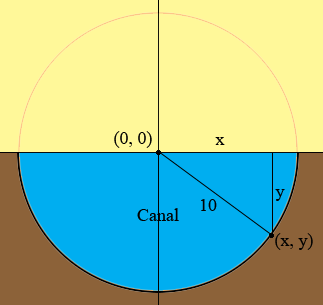SEARCH HOMEMath Central Quandaries & QueriesQuestion from Connor, a student: a canal with cross section a semicircle is 10m deep at the centre. Find an equation for the semicircle and use it to find the depth 4m from the edge wateva help you give would be much appreciated thank youHi Connor,

Since the cross section is a semicircle and it is 10 a deep at the centre, the radius of the circle is 10 m. Thus every point on the surface of the canal is 10 m from the centre. To write an equation I am going to place the centre of the circle at the origin in the Cartesian plane. Thus the centre has coordinates (0, 0).In my diagram I placed a point (x, y) on the surface of the canal and drew lines to construct a right triangle with sides of length x and y metres and hypotenuse 10 metres. What does Pythagoras theorem say about this triangle? This gives you a relationship between x and y but the requirement that (x, y) be on the lowe semicircle gives the further restriction that y ≤ 0.

Can you complete the problem now?

If you need more help write back,
PennyMath Central is supported by the University of Regina and The Pacific Institute for the Mathematical Sciences.